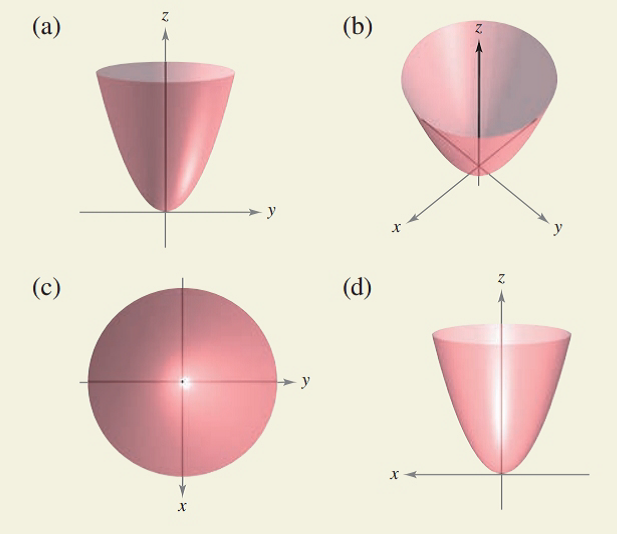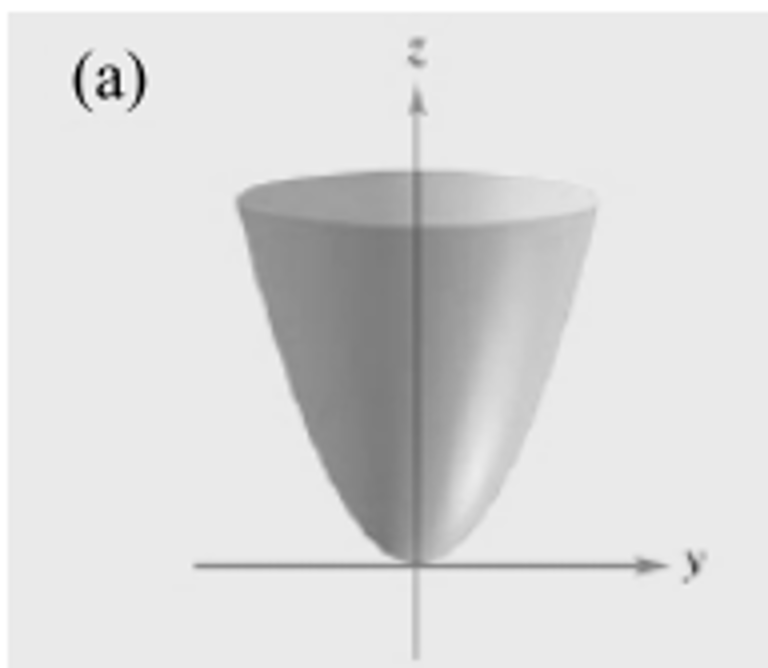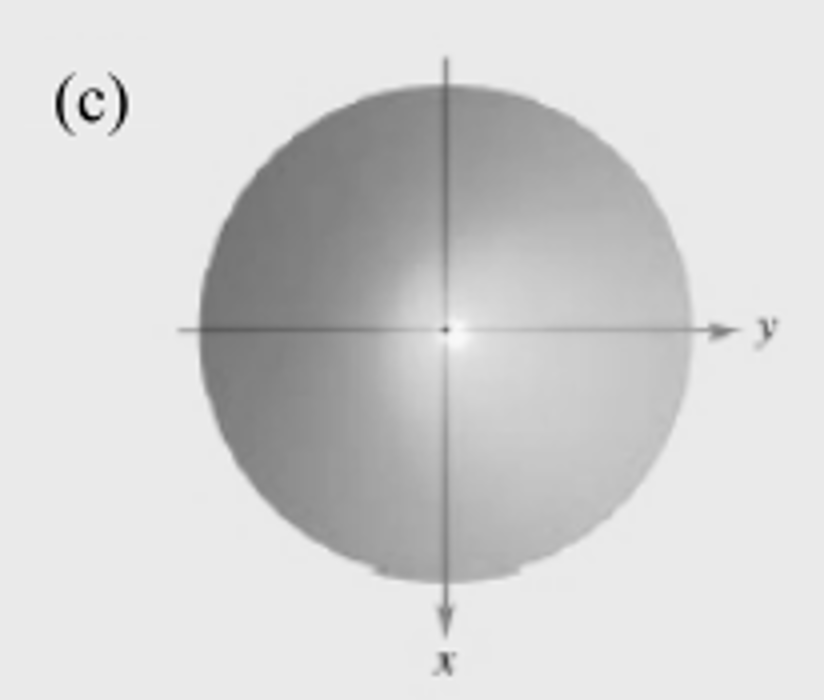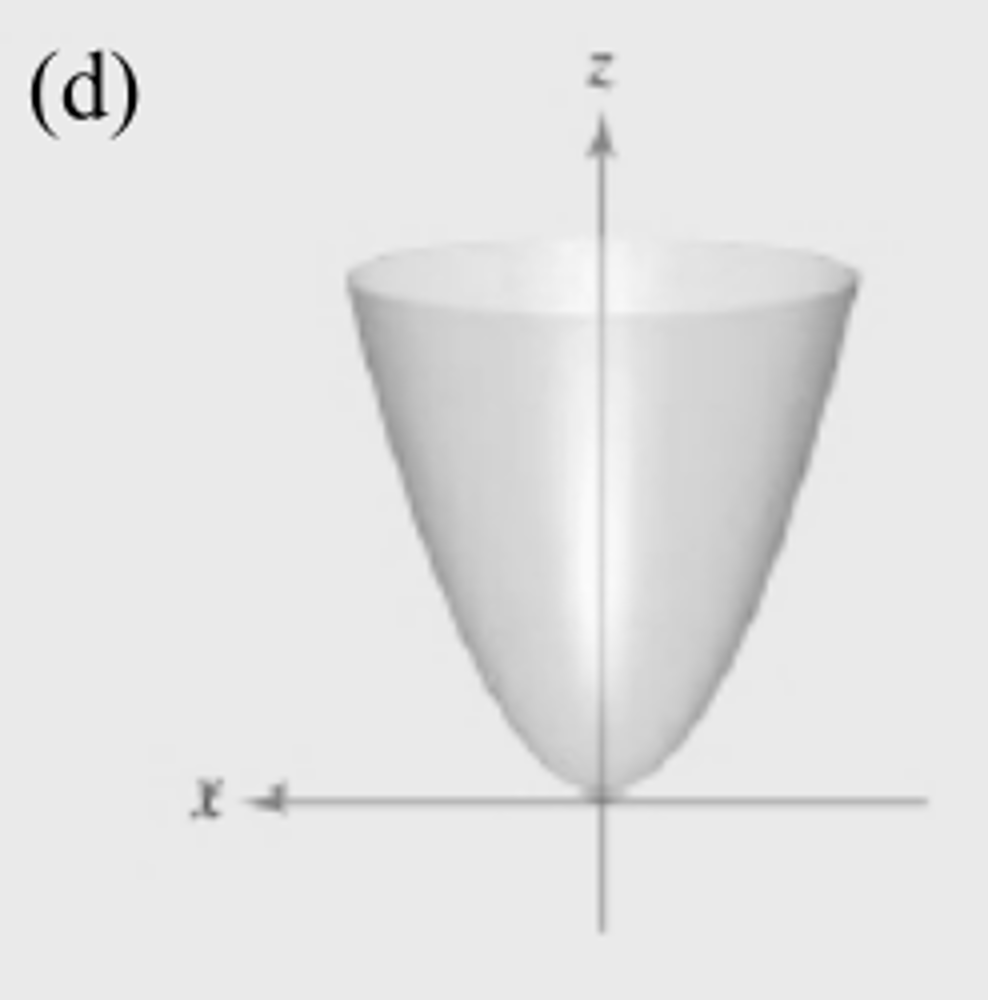Chapter 7.2, Problem 50E### Calculus: An Applied Approach (Min...

10th Edition
Ron Larson
ISBN: 9781305860919

#### Solutions

Chapter
Section### Calculus: An Applied Approach (Min...

10th Edition
Ron Larson
ISBN: 9781305860919
Textbook Problem
1 views

# HOW DO YOU SEE IT? The four figures are graphs of the quadric surface z = x 2 + y 2 . Match each of the four graphs with the point in space from which the paraboloid is viewed. The four points are (0, 0, 20), (0, 20, 0), (20, 0, 0), and (10, 10, 20).a)

To determine

The correct point in space from which the given graph of paraboloid z=x2+y2 is viewed.Explanation

Given Information:

The quadric surface equation is z=x2+y2 and the points in space from which the paraboloid is viewed are (0,0,20),(0,20,0),(20,0,0) and (10,10,20) and the graph is,

b)

To determine

The correct point in space from which the given graph of paraboloid z=x2+y2 is viewed.c)

To determine

The correct point in space from which the given graph of paraboloid z=x2+y2 is viewed.d)

To determine

The correct point in space from which the given graph of paraboloid z=x2+y2 is viewed.### Still sussing out bartleby?

Check out a sample textbook solution.

See a sample solution

#### The Solution to Your Study Problems

Bartleby provides explanations to thousands of textbook problems written by our experts, many with advanced degrees!

Get Started

#### In Exercises 73-80, find the indicated limits, if they exist. 73. limx3x+2x5

Applied Calculus for the Managerial, Life, and Social Sciences: A Brief Approach

#### For Problems 11-18, evaluate each expression. (3)(2)(15)(5)(8)(4)(10)

Mathematical Applications for the Management, Life, and Social Sciences

#### Let and g(x) = x + 3. Then (g ∘ h)(x) = ______.

Study Guide for Stewart's Single Variable Calculus: Early Transcendentals, 8th